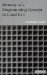# Exercises8.1 Consider the following C++ class:

` class A {    public:      int a;      char b;      A() { a = 0; b = '  class A { public: int a; char b; A() { a = 0; b = '\0'; } };//end class A  '; }    };//end class A `

Is it a problem that this class does not have a destructor? Is it a problem that the class does not have a copy constructor? Is it a problem that the class does not have an assignment?

8.2 Consider a C++ class:

` class A {    public:      B* b;      A() { b = new B(1,2); }  ~  A() { delete b; }      void* new(size_t size) {        ...      }    };//end class A `

When an object of class A is being constructed , which new is being used to create the object of class B : the class- A specific new , or the class- B specific new , or the global new ?

8.3 What is wrong with the following class, and what kind of problems might it cause during execution?

` class A {    public:     A() { string = 0; }     A(char* x) {      string = new char[strlen(x)+1];       strcpy(string,x);     }  ~  A() { if (string) free(string); }    A& operator= (const A& a) {     string = new char[strlen(a.string)+1];     strcpy(string,a.string);     return *this;    } `

8.4 The C++ compiler is complaining about "no appropriate default constructor for class A" but points to the Bb; statement. Why? How would you fix this problem?

` class A {    public:     char string;     A() { strcpy(string,"good bye"); }     A(char* s) { strcpy(string,s); }    };//end class A    class B : public A {    public:     int x;     B(char* s) : A(s) { }    };//end class B    int main()    {      A a("hello");      printf("%s\n",a.string);      B b;      printf("%s\n",b.string);      return 0;    } `

8.5 The following program is leaking memory. Find where and correct it.

` #include <iostream>    extern "C" {      #include <stdio.h>      #include <string.h>      #include <stdlib.h>    }    class A {    public:      char* string;      A(char* s) { string = strdup(s); }      A& operator=(const A& a) {        string = a.string;        return *this;      }    };//end class A    int main()    {      A a("hello");      printf("%s\n",a.string);      A b("good bye");      printf("%s\n",b.string);      b=a;      printf("%s\n",b.string);      return 0;    } `

8.6 Having used placement- new in our program, we should also define a corresponding placement- delete . Can we call it explicitly within our program?

8.7 Write a C++ program in which an object is created that has some static data, some data on the stack, and some data on the heap. Do not peek at the next exercise. Designing programs like this is not advisable in real life!

8.8 What will happen in the following C++ program if the display statement in main() is uncommented and the program is recompiled and executed? Can you describe the output?

` class A {    public:      char* heap_string;      char* stack_string;      A() { heap_string = stack_string = 0; }      void AttHStr(char* x) { heap_string = x; }      void AttSStr(char* x) { stack_string = x; }      friend ostream& operator << (ostream& os,const A& a) {        os << "heap string=" << a.heap_string << "\n";        os << "stack string=" << a.stack_string << "\n";        return os;      }    };    Aa;    void doit()    {      char s[] = "..stack..";      a.AttSStr(s);      cout << a << '\n';    }    int main()    {      a.AttHStr(strdup("..heap.."));      doit();      //cout << a << '\n';      return 0;    } `Memory as a Programming Concept in C and C++
ISBN: 0521520436
EAN: 2147483647
Year: 2003
Pages: 64

Similar book on Amazon

flylib.com © 2008-2017.
If you may any questions please contact us: flylib@qtcs.net